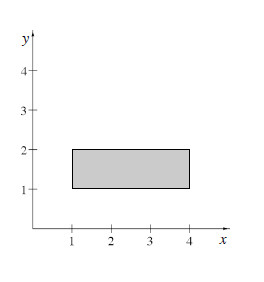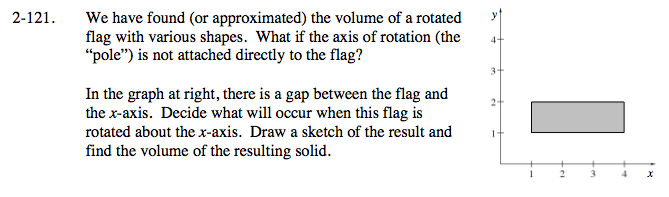### Home > CALC > Chapter 2 > Lesson 2.3.2 > Problem2-121

2-121.
1. We have found (or approximated) the volume of a rotated flag with various shapes. What if the axis of rotation (the "pole") is not attached directly to the flag?2. In the graph above, there is a gap between the flag and the x-axis. Decide what will occur when this flag is rotated about the x-axis. Draw a sketch of the result and find the volume of the resulting solid. To help you visualize this, use the 2-121 eTool (Desmos). Homework Help ✎A large cylinder, with a hollow cylindrical hole in the center.

Compute two volumes and subtract: a large solid cylinder − the cylindrical hole.

Both cylinders have a height of 3.

The large cylinder has a radius of 2. The small cylinder has a radius of 1.

Use the eTool below to visualize the flag.
Click the link at right for the full version of the eTool: Calc 2-121 HW eTool